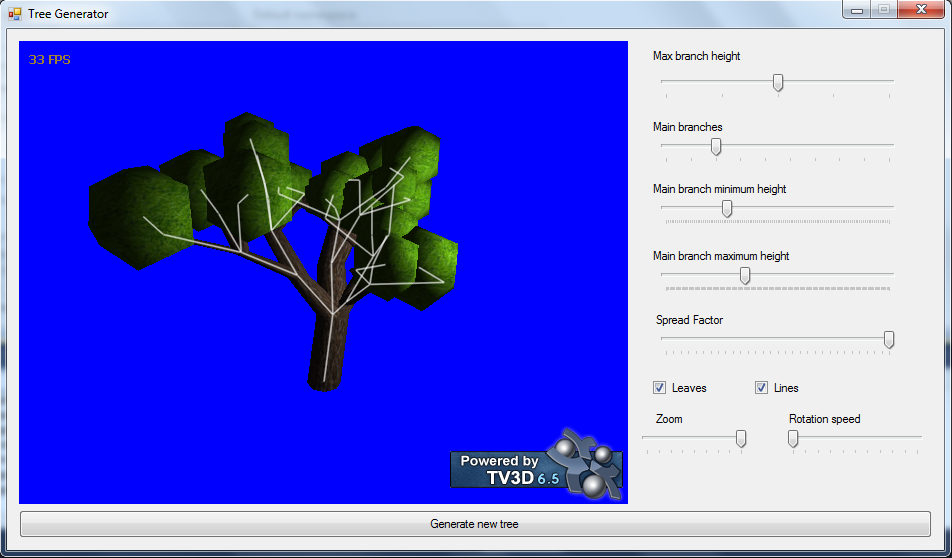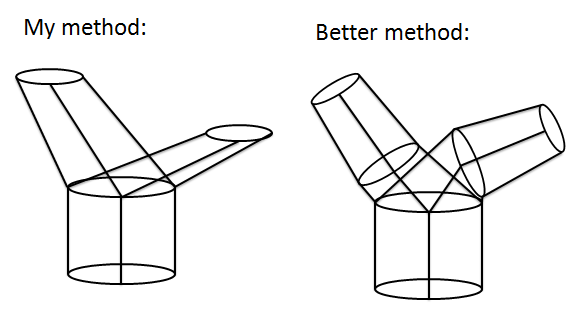# Procedurally generated tree(With source)

I was bored one day so I sat down and tried making a random tree generator. Essentially it’s extremely simple, you basically have this:

```struct Tree
{
int x, y, z;
Tree[] subTrees;
}```

It’s a root which defines a point in R3, a thickness and branches connected to it. From this structure I can generate a tree by recursively traversing the structure, building cylinders as I go:

```AddCylinder(int x1, int  y1, int  z1,
int  x2, int  y2, int  z2,
float w1, float w2, 3DModel model)
{
//Construct cylinder from (x1,y1,z1) with raidus w1 to (x2,y2,z2) with radius w2
}

BuildTree(Tree node, 3DModel model)
{
foreach(Tree subTree in node.subTrees)
{
subTree.x, subTree.y, subTree.z,
BuildTree(subTree, model)
}
}```

The interesting part of all this is finding an algorithm which generates cool trees. My algorithm was kind of half-assed, I have a much better idea now than I did when I was motivated to implement it. I was thinking of having three cases and then choosing between them: “Stop here”, “Continue growing” or “Branch out”. Then it would be a simple problem of choosing what would be best at any given time. My current algorithm is implemented like “I just want to see if it works”.(Note that the “leaves” are icosahedrons stolen from my first post)

As you can see the approach I have to constructing the cylinders is not as great as it could have been. The ends are always on an XY plane, or aligned with the floor. I could’ve rotated them so they were facing the direction they’re growing in and then connected them with an extra cylinder at each node:The generator has the potential of building really realistic trees by adding a lot more small branches and adding a lot of small leave sprites instead of big spheres added to the end of each branch.

It’s written in C# using .NET 3.5 and TrueVision3D(DLL included). Here’s the source: